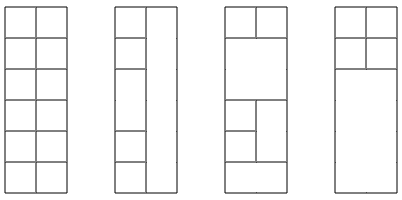CSES - Counting Towers
• Time limit: 1.00 s
• Memory limit: 512 MB
Your task is to build a tower whose width is $2$ and height is $n$. You have an unlimited supply of blocks whose width and height are integers.

For example, here are some possible solutions for $n=6$:Given $n$, how many different towers can you build? Mirrored and rotated towers are counted separately if they look different.

Input

The first input line contains an integer $t$: the number of tests.

After this, there are $t$ lines, and each line contains an integer $n$: the height of the tower.

Output

For each test, print the number of towers modulo $10^9+7$.

Constraints
• $1 \le t \le 100$
• $1 \le n \le 10^6$
Example

Input:
3
2
6
1337

Output:
8
2864
640403945